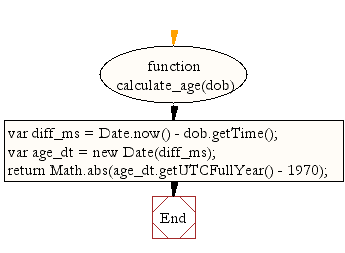# JavaScript: Calculate age

## JavaScript Datetime: Exercise-18 with Solution

Write a JavaScript program to calculate age.

Test Data:
console.log(calculate_age(new Date(1982, 11, 4)));
35
console.log(calculate_age(new Date(1962, 1, 1)));
56

Sample Solution:-

HTML Code:

``````<!DOCTYPE html>
<html>
<meta charset="utf-8">
<title>JavaScript program to calculate age</title>
<body>

</body>
</html>
```
```

JavaScript Code:

``````function calculate_age(dob) {
var diff_ms = Date.now() - dob.getTime();
var age_dt = new Date(diff_ms);

return Math.abs(age_dt.getUTCFullYear() - 1970);
}

console.log(calculate_age(new Date(1982, 11, 4)));

console.log(calculate_age(new Date(1962, 1, 1)));
```
```

Sample Output:

```35
56
```

Flowchart:Live Demo:

See the Pen JavaScript - Calculate age-date-ex-18 by w3resource (@w3resource) on CodePen.

Improve this sample solution and post your code through Disqus

What is the difficulty level of this exercise?

﻿

## JavaScript: Tips of the Day

```const user = { name: 'Owen', age: 21 };